# xSYR2K (Symmetric Matrix Rank-2k Update) VI

LabVIEW 2012 GPU Analysis Toolkit Help

Edition Date: June 2012

Part Number: 373575A-01

»View Product InfoDownload Help (Windows Only)

Owning Palette: LVCUBLAS

Requires: GPU Analysis Toolkit

Calculates the rank-2k update of a symmetric matrix. When you wire data to A in, B in (if available), and C, this VI automatically selects the first available instance.

To use a different instance, you must manually select the polymorphic instance you want to use.

Use the pull-down menu to select an instance of this VI.

 Select an instance xSYR2K (Symmetric Matrix Rank-2k Update) (aAA' + conj(a)AA' + bC)xSYR2K (Symmetric Matrix Rank-2k Update) (aA'A + conj(a)A'A + bC)xSYR2K (Symmetric Matrix Rank-2k Update) (aAB' + conj(a)BA' + bC)xSYR2K (Symmetric Matrix Rank-2k Update) (aA'B + conj(a)B'A + bC)

## xSYR2K (Symmetric Matrix Rank-2k Update) (aAA' + conj(a)AA' + bC)

The connector pane displays the default data types for this polymorphic instance.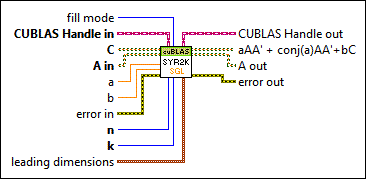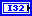fill mode specifies the triangular portion of the matrix A in the calculation.

 0 CUBLAS_FILL_MODE_LOWER (default) 1 CUBLAS_FILL_MODE_UPPERCUBLAS Handle in specifies the initialized CUBLAS library to use for the BLAS calculation. For example, you can wire the CUBLAS Handle output from the Initialize Library VI to specify the CUBLAS handle to the CUBLAS library you already initialized. This input also determines the device that executes the function.C specifies the symmetric matrix C stored on the device. This input specifies a class that can contain the following data types:

• Single-precision, floating-point numeric
• Double-precision, floating-point numeric
• Complex single-precision, floating-point numeric
• Complex double-precision, floating-point numericA in specifies the matrix A stored on the device. This input specifies a class that can contain the following data types:

• Single-precision, floating-point numeric
• Double-precision, floating-point numeric
• Complex single-precision, floating-point numeric
• Complex double-precision, floating-point numeric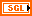a represents alpha and specifies the scalar operand for the product alpha*A*A' and conj(alpha)*A*A'. The default is 1.b represents beta and specifies the scalar operand in the product beta*C. The default is 0.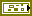error in describes error conditions that occur before this node runs. This input provides standard error in functionality.n specifies the number of rows to use in matrix A.k specifies the number of columns to use in matrix A'.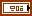leading dimensions specifies the column dimension to index consecutive rows. Use lda, ldb, and ldc for A, A, and C, respectively.CUBLAS Handle out returns the handle that defines the BLAS operation.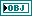aAA' + conj(a)AA'+bC returns a matrix with the same dimensions as C. For the elements of the first n rows and n columns of the triangular component defined by fill mode, aAA' + conj(a)AA'+bC returns the result of the calculation. For any remaining elements, aAA' + conj(a)AA'+bC returns the value of the element with the same index in C.A out returns the triangular matrix A stored on the device.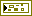error out contains error information. This output provides standard error out functionality.

## xSYR2K (Symmetric Matrix Rank-2k Update) (aA'A + conj(a)A'A + bC)

The connector pane displays the default data types for this polymorphic instance.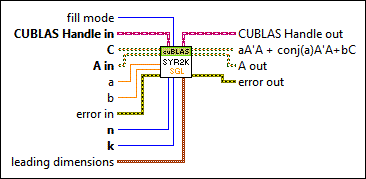fill mode specifies the triangular portion of the matrix A in the calculation.

 0 CUBLAS_FILL_MODE_LOWER (default) 1 CUBLAS_FILL_MODE_UPPERCUBLAS Handle in specifies the initialized CUBLAS library to use for the BLAS calculation. For example, you can wire the CUBLAS Handle output from the Initialize Library VI to specify the CUBLAS handle to the CUBLAS library you already initialized. This input also determines the device that executes the function.C specifies the symmetric matrix C stored on the device. This input specifies a class that can contain the following data types:

• Single-precision, floating-point numeric
• Double-precision, floating-point numeric
• Complex single-precision, floating-point numeric
• Complex double-precision, floating-point numericA in specifies the matrix A stored on the device. This input specifies a class that can contain the following data types:

• Single-precision, floating-point numeric
• Double-precision, floating-point numeric
• Complex single-precision, floating-point numeric
• Complex double-precision, floating-point numerica represents alpha and specifies the scalar operand for the product alpha*A'*A and conj(alpha)*A'*A. The default is 1.b represents beta and specifies the scalar operand in the product beta*C. The default is 0.error in describes error conditions that occur before this node runs. This input provides standard error in functionality.n specifies the number of rows to use in matrix A'.k specifies the number of columns to use in matrix A.leading dimensions specifies the column dimension to index consecutive rows. Use lda, ldb, and ldc for A, A, and C, respectively.CUBLAS Handle out returns the handle that defines the BLAS operation.aA'A + conj(a)A'A+bC returns a matrix with the same dimensions as C. For the elements of the first n rows and n columns of the triangular component defined by fill mode, aA'A + conj(a)A'A+bC returns the result of the calculation. For any remaining elements, aA'A + conj(a)A'A+bC returns the value of the element with the same index in C.A out returns the triangular matrix A stored on the device.error out contains error information. This output provides standard error out functionality.

## xSYR2K (Symmetric Matrix Rank-2k Update) (aAB' + conj(a)BA' + bC)

The connector pane displays the default data types for this polymorphic instance.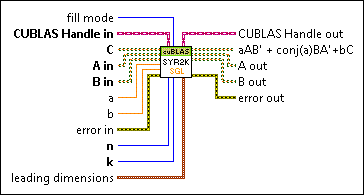fill mode specifies the triangular portion of the matrix A in the calculation.

 0 CUBLAS_FILL_MODE_LOWER (default) 1 CUBLAS_FILL_MODE_UPPERCUBLAS Handle in specifies the initialized CUBLAS library to use for the BLAS calculation. For example, you can wire the CUBLAS Handle output from the Initialize Library VI to specify the CUBLAS handle to the CUBLAS library you already initialized. This input also determines the device that executes the function.C specifies the symmetric matrix C stored on the device. This input specifies a class that can contain the following data types:

• Single-precision, floating-point numeric
• Double-precision, floating-point numeric
• Complex single-precision, floating-point numeric
• Complex double-precision, floating-point numericA in specifies the matrix A stored on the device. This input specifies a class that can contain the following data types:

• Single-precision, floating-point numeric
• Double-precision, floating-point numeric
• Complex single-precision, floating-point numeric
• Complex double-precision, floating-point numericB in specifies the matrix B stored on the device. This input specifies a class that can contain the following data types:

• Single-precision, floating-point numeric
• Double-precision, floating-point numeric
• Complex single-precision, floating-point numeric
• Complex double-precision, floating-point numerica represents alpha and specifies the scalar operand for the product alpha*A*B' and conj(alpha)*B*A'. The default is 1.b represents beta and specifies the scalar operand in the product beta*C. The default is 0.error in describes error conditions that occur before this node runs. This input provides standard error in functionality.n specifies the number of rows to use in matrix B.k specifies the number of columns to use in matrix A.leading dimensions specifies the column dimension to index consecutive rows. Use lda, ldb, and ldc for A, B, and C, respectively.CUBLAS Handle out returns the handle that defines the BLAS operation.aAB' + conj(a)BA'+bC returns a matrix with the same dimensions as C. For the elements of the first n rows and n columns of the triangular component defined by fill mode, aAB' + conj(a)BA'+bC returns the result of the calculation. For any remaining elements, aAB' + conj(a)BA'+bC returns the value of the element with the same index in C.A out returns the triangular matrix A stored on the device.B out returns the matrix B stored on the device.error out contains error information. This output provides standard error out functionality.

## xSYR2K (Symmetric Matrix Rank-2k Update) (aA'B + conj(a)B'A + bC)

The connector pane displays the default data types for this polymorphic instance.fill mode specifies the triangular portion of the matrix A in the calculation.

 0 CUBLAS_FILL_MODE_LOWER (default) 1 CUBLAS_FILL_MODE_UPPERCUBLAS Handle in specifies the initialized CUBLAS library to use for the BLAS calculation. For example, you can wire the CUBLAS Handle output from the Initialize Library VI to specify the CUBLAS handle to the CUBLAS library you already initialized. This input also determines the device that executes the function.C specifies the symmetric matrix C stored on the device. This input specifies a class that can contain the following data types:

• Single-precision, floating-point numeric
• Double-precision, floating-point numeric
• Complex single-precision, floating-point numeric
• Complex double-precision, floating-point numericA in specifies the matrix A stored on the device. This input specifies a class that can contain the following data types:

• Single-precision, floating-point numeric
• Double-precision, floating-point numeric
• Complex single-precision, floating-point numeric
• Complex double-precision, floating-point numericB in specifies the matrix B stored on the device. This input specifies a class that can contain the following data types:

• Single-precision, floating-point numeric
• Double-precision, floating-point numeric
• Complex single-precision, floating-point numeric
• Complex double-precision, floating-point numerica represents alpha and specifies the scalar operand for the product alpha*A'*B and conj(alpha)*B'*A. The default is 1.b represents beta and specifies the scalar operand in the product beta*C. The default is 0.error in describes error conditions that occur before this node runs. This input provides standard error in functionality.n specifies the number of rows to use in matrix A.k specifies the number of columns to use in matrix B.leading dimensions specifies the column dimension to index consecutive rows. Use lda, ldb, and ldc for A, B, and C, respectively.CUBLAS Handle out returns the handle that defines the BLAS operation.aA'B + conj(a)B'A+bC returns a matrix with the same dimensions as C. For the elements of the first n rows and n columns of the triangular component defined by fill mode, aA'B + conj(a)B'A+bC returns the result of the calculation. For any remaining elements, aA'B + conj(a)B'A+bC returns the value of the element with the same index in C.A out returns the triangular matrix A stored on the device.B out returns the matrix B stored on the device.error out contains error information. This output provides standard error out functionality.

## xSYR2K (Symmetric Matrix Rank-2k Update) Details

For more information on how to use this VI, refer to the Designing the Block Diagram to Compute on a GPU Device topic.

For more information about the CUBLAS library and BLAS operations, refer to the NVIDIA GPU Computing Documentation website at nvidia.com and download the CUBLAS Library User Guide.

Refer to the BLAS (Basic Linear Algebra Subprograms) website at netlib.org for more information on BLAS functions.